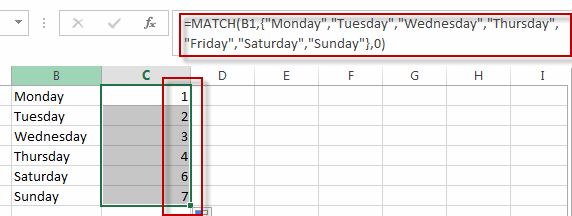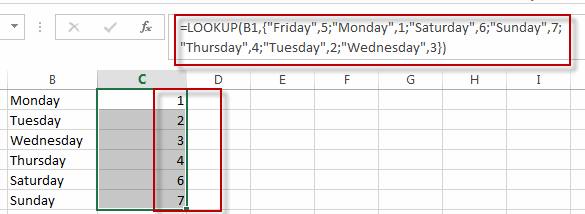# Convert Weekday Name to Number

This post will guide you how to convert weekday string to number in excel. How do I convert weekday name to number with a formula in excel. How to convert day of week to number in excel. Is there an easy way to convert a weekday string which is either “Monday” or “Tuesday” into a number in excel.

## Convert Weekday Name to Number

Assuming that you have list of data B1:B7 that contain weekday names, you want to convert all weekday names to numbers, how to achieve it.

You can use a formula based on the MATCH function to achieve the result. Just like this formula:

`=MATCH(B1,{"Monday","Tuesday","Wednesday","Thursday","Friday","Saturday","Sunday"},0)`

Type this formula into the formula box of one blank cell, such as: Cell C1, then press Enter key in your keyboard, and drag the AutoFill Handler over other cells to apply this formula to convert weekday string to numbers.You will see all weekday names in range B1:B7 have been converted to numbers in range C1:C7.

Or you can also use another complex formula based on the LOOKUP function to convert weekday names to numbers in excel. Like this:

`=LOOKUP(A1,{"Monday",1;"Friday",5;"Saturday",6;"Sunday",7;"Thursday",4;"Tues  day",2;"Wednesday",3})`### Related Functions

• Excel LOOKUP function
The Excel LOOKUP function will search a value in a vector or array.The syntax of the LOOKUP function is as below:= LOOKUP (lookup_value, lookup_vector, [result_vector])…
• Excel MATCH  function
The Excel MATCH function search a value in an array and returns the position of that item.The MATCH function is a build-in function in Microsoft Excel and it is categorized as a Lookup and Reference Function.The syntax of the MATCH function is as below:= MATCH  (lookup_value, lookup_array, [match_type])….